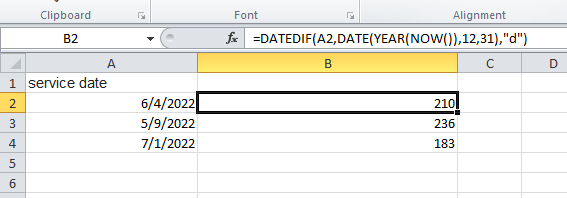# Calculate date difference in excel and update corresponding column

Hi Everyone,

My requirement is i have an excel with many columns

1. I add a column to the excel called YOS
2. Then i have to calculate date difference b/w other column in same excel called ‘service date’ with last day of year and update YOS for corresponding rows

'service date ’ has dates in dd/MM/yyyy format and excel has 5k records normally so for each will consume time , any solution for this query

you can try excel as db and try this query

``````SELECT [service date],DateDiff("d", [service date],DateAdd("d", -1, DateSerial(Year(Date())+1,1,1)) ) As [YOS]  from Sheet1;
``````

first we find the last date of year , then we find out the difference of days between “service date” and the last date

or in excel you can use this formula

=DATEDIF(A2,DATE(YEAR(NOW()),12,31),“d”)

this will give the days difference

Hello @kavya.s16 ,

You can refer to the below post. Same like that you can create the flow, but while writing the vb script, you can use the expression below.

DateDiff(DateInterval, row(“service date”),DateTime.Now.ToString(“dd/MM/yyyy” )

if you want to get day difference.
DateDiff(DateInterval.Day, row(“service date”),DateTime.Now.ToString(“dd/MM/yyyy” )

1 Like

this is giving #value error @saurabhB
how to use the excel formula in uipath

could u pls elaborate? @saurabhBI have used the excel formula using the above data in image

For excel as db setup check this link How To Use Excel As Database – In UiPath – ExcelCult
I had written some incorrect syntax In the SQL query, pl try this (we need to add \$ sign with the sheet name)

``````SELECT [service date],DateDiff("d", [service date],DateSerial(Year(Date()),12,31) ) As [YOS]  from [Sheet1\$]
``````